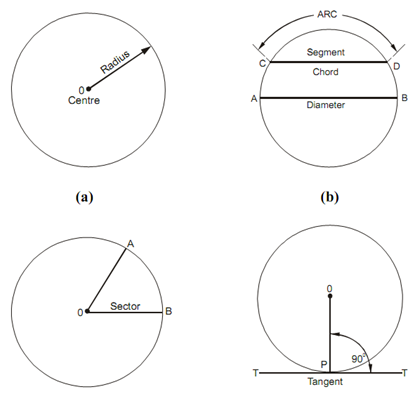## Circle and Curves Assignment Help

Assignment Help: >> Geometrical Construction - Circle and Curves

Circle and Curves:

A circle is a plane figure bounded via one line identified as circumference that is always equidistant from the fixed point identified as center. The fixed distance from the center to the circumference is called radius in diagram (a). The diameter of a circle is a straight line drawn via the center and meeting the circumference at both the ends. The diameter is two times the radius. An arc is any part of the circumference of a circle as in diagram (b). A chord is a straight line joining any two points on the circumference in diagram (b). A segment is a part of a circle that is bounded with an arc and a chord as displayed in diagram (b). A sector is a part of a circle that is bounded with an arc and two radii in diagram. A tangent to a circle is a straight line that touches the circumference of the circle but does not cut it as in diagram. Concentric circles are those have the same centre however different radii. Eccentric circles are those have different centers.Divide a Circle into Five or Seven Equal Parts Dividing a Circle into Four or Eight Equal Parts Dividing a Circle into Three - Six and Twelve Equal Parts Finding the Center of a given Circle#### Assured A++ Grade

Get guaranteed satisfaction & time on delivery in every assignment order you paid with us! We ensure premium quality solution document along with free turntin report!

All rights reserved! Copyrights ©2019-2020 ExpertsMind IT Educational Pvt Ltd